# SUMMARY OF TESTS SUMMARY OF TESTS Series Tests

• Slides: 25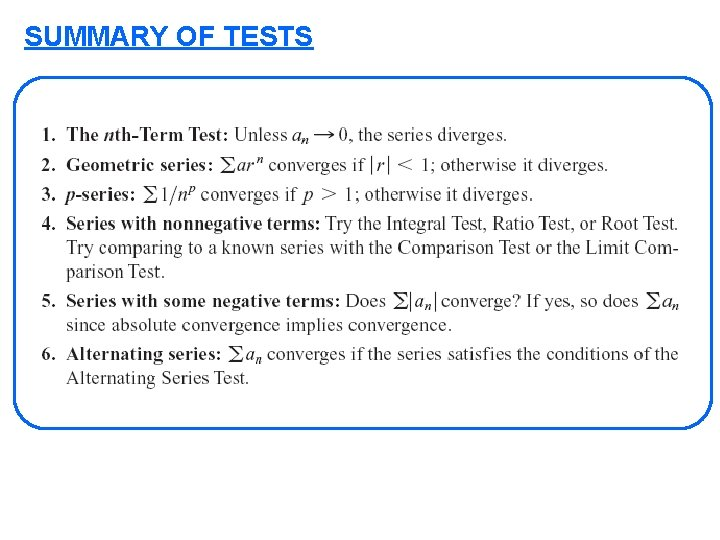SUMMARY OF TESTSSUMMARY OF TESTS Series Tests Special Series: 1) Divergence Test 1) Geometric Series 2) Integral Test 2) Harmonic Series 3) Comparison Test 3) Telescoping Series 4) Limit Compar Test 4) Alter Harmonic 5) Ratio Test 5) p-series 6) Root Test 6) Alternating p-series 7) Alter Series TestTypes of questions in the exam 1) Determine whether convg or divg AC, CC 2) Find the sum s 4 -types 5) Partial sums and their properties 4) How many terms are needed within errorPART-1: Series with positive terms 1) Divg Test 2) factorial: ratio test comp+lim comp 3) power of n: root test 4) easy to integrate: integral test 5) similar to geometric: try comp+lim comp 6) Similar to p-series: try comp+lim comp STRATEGY FOR TESTING SERIES PART-2: Alternating Series 1) Study (use PART-1) convg divg use alt. -Test AC convg CC divg PART-3: Series with some negative terms 1) Study convg AC (use PART-1) divg REMARK: For multiple-choice-question: before you start read the alternatives first. It guides you to which tests you need to use.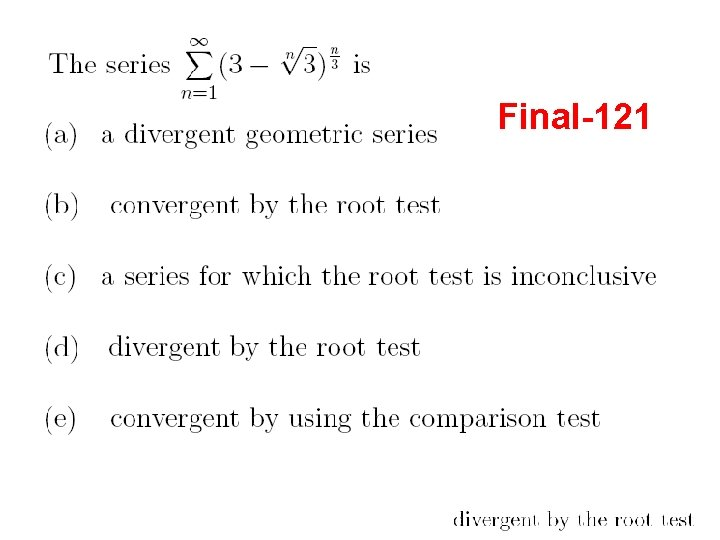Final-121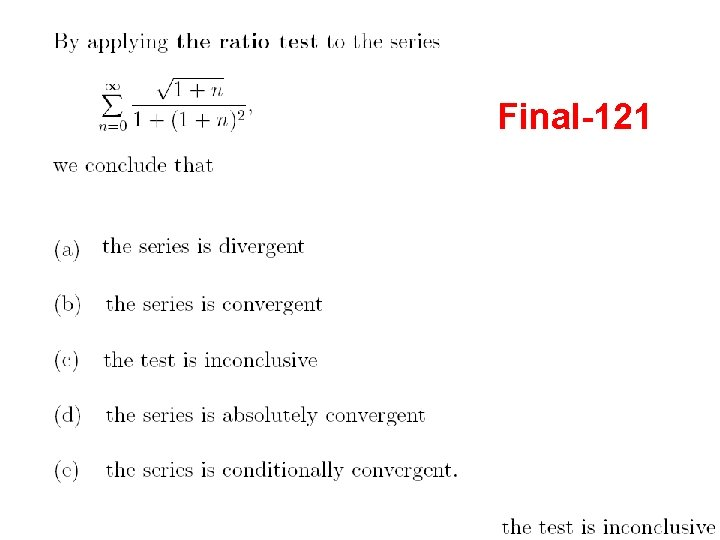Final-121Final-122Final-121Final-131Final-131Final-131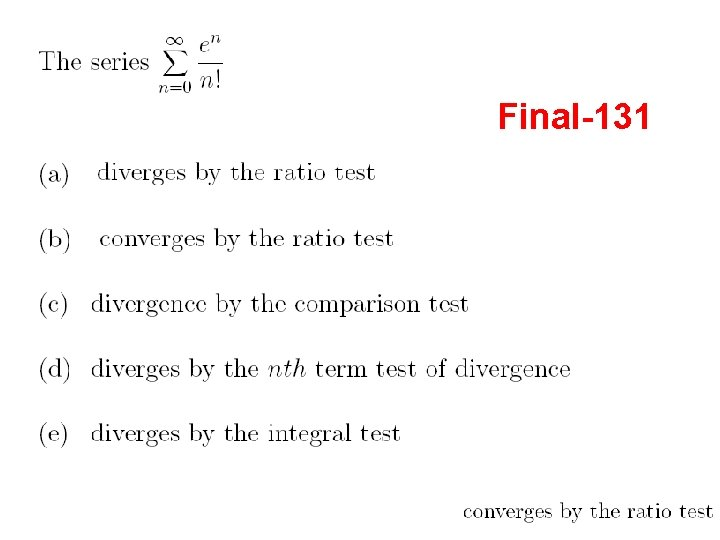Final-131Final-122Final-122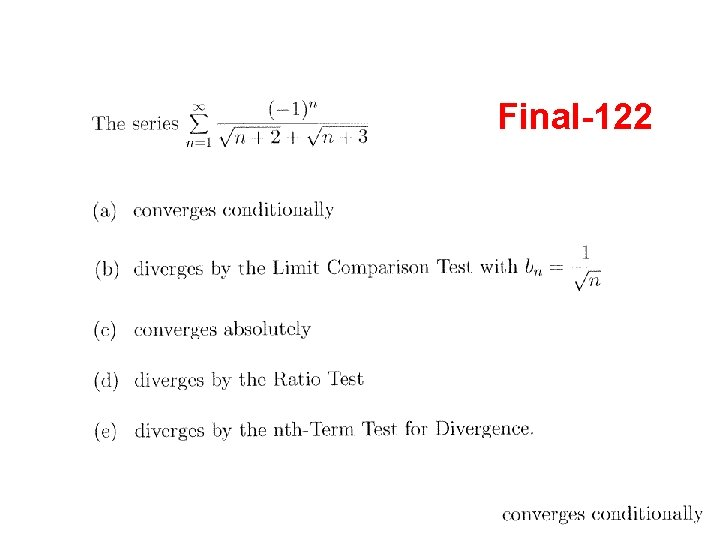Final-122Final-122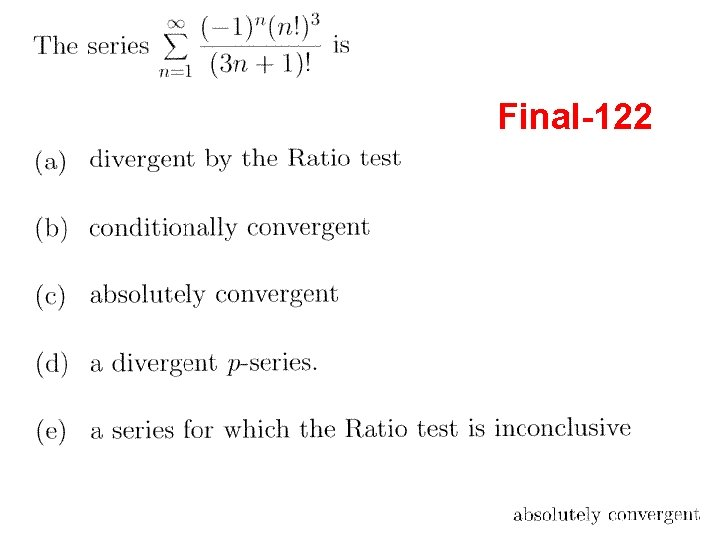Final-122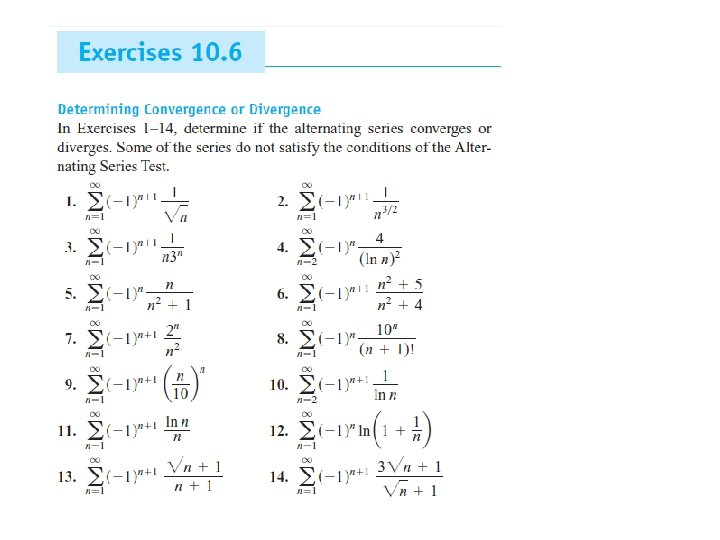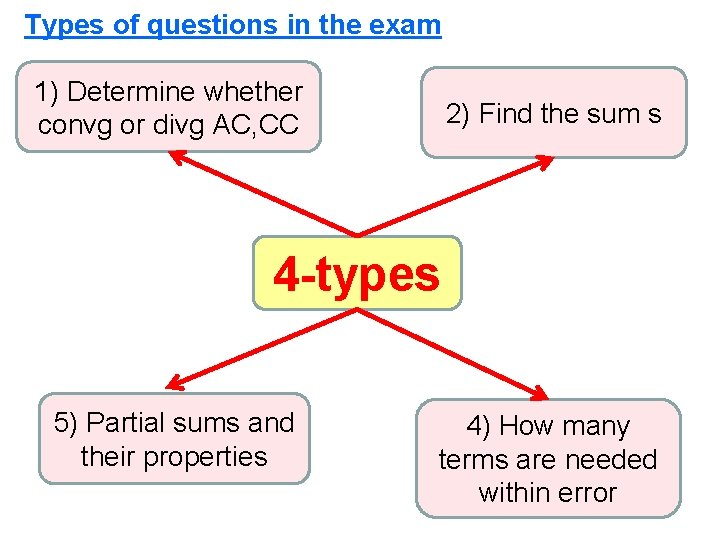Types of questions in the exam 1) Determine whether convg or divg AC, CC 2) Find the sum s 4 -types 5) Partial sums and their properties 4) How many terms are needed within error4) How many terms are needed within error PART-1: Series with positive terms PART-2: Alternating Series